# Example of Minimization of Deterministic Finite Automata (DFA)

Minimization of DFA (Table Filling Method or Myhill-Nerode Theorem)

Steps:

1. Draw a table for all pairs of states (P, Q)
2. Mark all pairs where Pϵ F and Q∉F
3. If there are any Unmarked pairs (P, Q) such that [δ(P, x),δ(Q, x)] is marked, then mark [P, Q] where ‘x’ is an input symbol. Repeat this until no more marking can be made.
4. Combine all the unmarked pairs and make them a single state in the minimized DFA.

Example: Minimize the following DFA using Table Filling Method.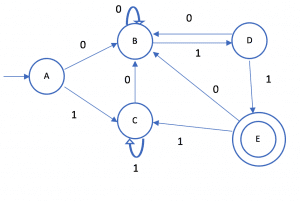Step 1: Draw a table for all pairs of states (P, Q)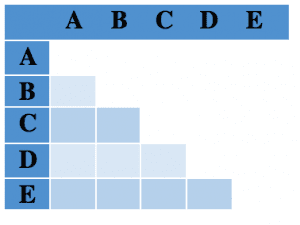Step 2: Mark all pairs where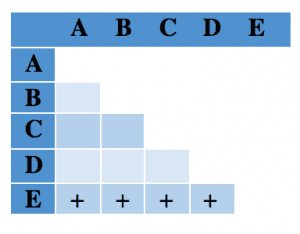Step 3: If there are any Unmarked pairs (P, Q) such that [δ(P, x),δ(Q, x)] is marked, then mark [P, Q] where ‘x’ is an input symbol. Repeat this until no more marking can be made.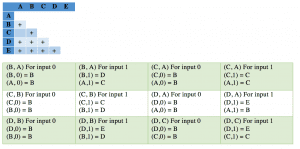Step 4 :(A, C), B, D, E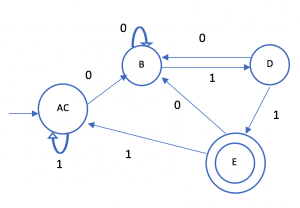## 1 comment

1.Shaikh Abdul razzaque says:

Yas sir

This site uses Akismet to reduce spam. Learn how your comment data is processed.